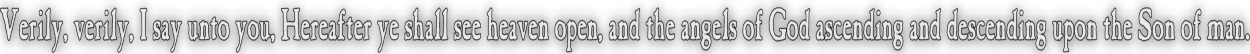None:
Polyps:
Strongs:MetaphysicsHartshorne's Modal Proof. Using the Modal operators N(x) and P(x) for "x is logically necessary" (i.e. correct) and "x is logically possible" respectively we immediately have ¬P(x) = N(¬x) and ¬N(¬x) = P(x). 1) G => N(G) Anselm's principle. 2) N(G) v ¬N(G) The Existence Of God is necessary, or not necessary, (excluded middle). 3) N(G) v N¬N(G) Becker's postulate: Modal status is always necessary. The status of something necessary is always necessary. 4) N(G) v N(¬G)   =>   N(G) v ¬P(G) Contrapositive of (1) applied. 5) P(G)  =  ¬N(¬G) Our postulate, the existence of perfection is not impossible. 6) N(G) Our result. (the left side of the "or" statement.) 7) N(G) => G Modal axiom 8) G God, a perfect being exists.Continue To Next Page Return To Section Start Return To Previous Page'